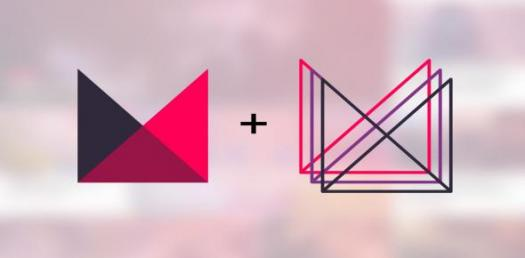# Polygon Property Test Quiz

10 Questions | Total Attempts: 325SettingsPolygons are shapes with two or more sides. They are made from lines that are joined together at certain angles. For a regular polygon, there are at least two sides that fuse to form a vertex. Some polygons have equal, interior angles while others do not. Also, exterior angles can be equal or not. Do you know so much about property of polygons?

Related Topics
• 1.
What is a polygon with five sides called?
• A.

Hexagon

• B.

Pentagon

• C.

Decagon

• D.

Heptagon

• 2.
Which of these is not a type of polygon?
• A.

Simple polygon

• B.

Complex polygon

• C.

Concave polygon

• D.

Convex polygon

• 3.
What type of polygon do not intersect themselves?
• A.

Simple polygon

• B.

Complex polygon

• C.

Concave polygon

• D.

Convex polygon

• 4.
What is the sum of interior angles in simple polygons?
• A.

180 degrees

• B.

120 degrees

• C.

90 degrees

• D.

100 degrees

• 5.
What are all convex polygons called?
• A.

Compound polygons

• B.

Convex polygons

• C.

Special polygons

• D.

Simple polygons

• 6.
What are polygons with equal edges called?
• A.

Isogonal figures

• B.

Equilateral figures

• C.

Tangential figures

• D.

Cyclic figures

• 7.
What is a polygons that has all sides perpendicular to each other called?
• A.

Tangential polygons

• B.

Isotoxal polygon

• C.

Regular polygon

• D.

Rectilinear polygons

• 8.
What is the sum of all angles forming a polygon?
• A.

90 degrees

• B.

360 degrees

• C.

180 degrees

• D.

270 degrees

• 9.
Which of these is not a variety of polygon?
• A.

Apeirogon

• B.

Skew polygon

• C.

Spherical polygon

• D.

Axial polygon

• 10.
What kind of polygons have algebraic properties?
• A.

Abstract polygon

• B.

Special polygon

• C.

Inscribed polygon

• D.

Tangential polygon